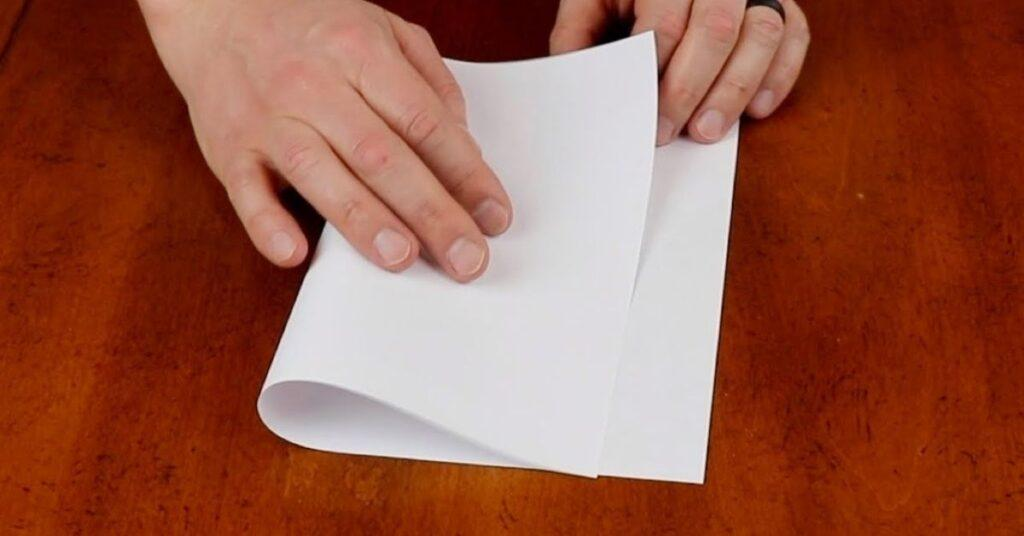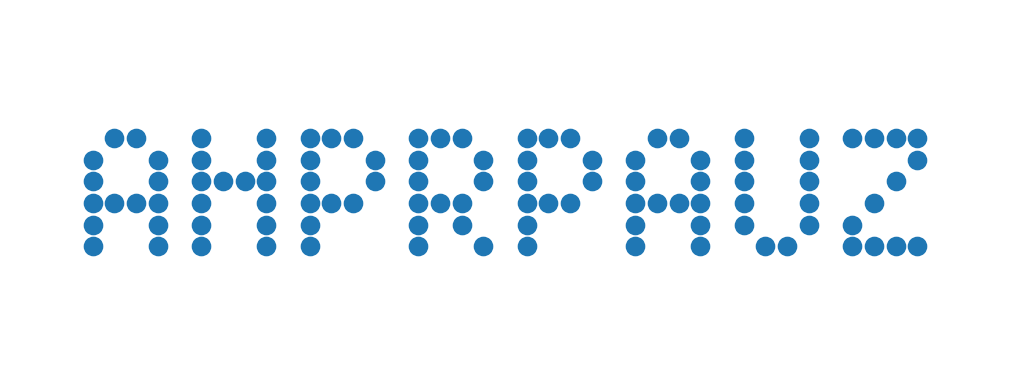# Learning Python with Advent of Code Walkthroughs

Dazbo's Advent of Code solutions, written in Python# Advent of Code 2021 - Day 13

## Problem Intro

Phew. Back to something a bit quicker to solve. Take a moment to appreciate this challenge. After this one, you wont see me using the word trivial again!!

We want to activate the sub’s thermal imaging system. But to activate it, we need a code from the instruction manual. (Anyone else nostalgic for 90s copy protection?) In the manual is a transparent sheet with random dots, and a set of instructions on how to fold the paper.

The imput looks like this:

``````6,10
0,14
9,10
0,3
10,4
4,11
...
fold along y=7
fold along x=5
``````
• The number pairs are the x,y coordinates of each dot on the paper; where 0,0 is the top.
• We’re told that dots will never appear on a fold line.
• When we fold, we’ll find that many dots from each side of the fold line will be overlapping.

## Part 1

We’re asked how many dots are visible, after performing the first fold instruction. (Remember that some dots will overlap.)

### Setup

Nothing new here. I’m using matplotlib because I want to do some visualisation later.

``````from dataclasses import dataclass
import logging
import os
import time
from matplotlib import pyplot as plt

SCRIPT_DIR = os.path.dirname(__file__)
INPUT_FILE = "input/input.txt"
# INPUT_FILE = "input/sample_input.txt"

logging.basicConfig(format="%(asctime)s.%(msecs)03d:%(levelname)s:%(name)s:\t%(message)s",
datefmt='%Y-%m-%d %H:%M:%S')
logger = logging.getLogger(__name__)
logger.setLevel(level=logging.DEBUG)
``````

### The solution

First, some basic dataclasses:

``````@dataclass
class Instruction:
""" Paper fold instruction """
axis: str   # x or y
val: int

@dataclass(frozen=True)
class Point:
x: int
y: int
``````

Note that we’ve made the `Point` class `frozen`. This makes instances of this class immutable and hashable. We need these `Point` objects to be hashable, because we’re going to store them in a `set` later.

We’ll now read in the data:

``````def process_data(data: str) -> tuple[set[Point], list]:
""" Input has n rows of x,y coords, then an empty line, then rows of instructions """

coords, _, instruction_lines = data.partition('\n\n')
dots = set()
for coord in coords.splitlines():    # e.g. [6, 10]
x,y = map(int, coord.split(","))

instructions = []
for line in instruction_lines.splitlines():
instr = line.replace("fold along ", "").split("=")
instructions.append(Instruction(instr, int(instr)))

return dots, instructions
``````

This splits the input data at the blank line, since all the lines before the blank line are points, and the lines after are fold instructions.

We convert the fold instructions into `Instruction` objects, just to make this easier to read and use later.

Now we’ll create a `Paper` class, that stores the current state of our folded transparent paper:

``````class Paper():
""" Represents transparent paper with dots at specified x,y locations.
The paper knows how to fold itself, given an instruction with an x or y value to fold along. """
def __init__(self, dots: set[Point]) -> None:
self._dots: set[Point] = dots

@property
def dot_count(self) -> int:
""" Total number of dots showing on the paper """
return len(self._dots)

def __str__(self) -> str:
""" Convert the dots to a printable string """
height = max(point.y for point in self._dots)
width = max(point.x for point in self._dots)

rows = []
for row in range(height+1):
row_str = ""
for col in range(width+1):
coord = Point(col, row)
row_str += "#" if coord in self._dots else " "

rows.append(row_str)

return "\n".join(rows)

def fold(self, instruction: Instruction):
""" Fold along a given axis.  Returns the union set of
numbers before the fold line, and the flip of the numbers after the fold line. """
assert instruction.axis in ('x', 'y'), "Instruction must be 'x' or 'y'"

before_fold = set()
after_fold = set()

if instruction.axis == 'x':    # fold vertical
before_fold = set(dot for dot in self._dots if dot.x < instruction.val)
after_fold = set(dot for dot in self._dots if dot.x > instruction.val)
folded = set(Point(instruction.val-(num.x-instruction.val), num.y) for num in after_fold)
else:   # fold horizontal
before_fold = set(dot for dot in self._dots if dot.y < instruction.val)
after_fold = set(dot for dot in self._dots if dot.y > instruction.val)
folded = set(Point(num.x, instruction.val-(num.y-instruction.val)) for num in after_fold)

self._dots = before_fold | folded
``````

• The `__init__()` method simply takes the `set` of points we read in from the input data.
• We have a `dot_count property` which returns the number of dots, i.e. by counting the number of members in the `_dots set`.
• We have a `__str__()` method which is called whenever we reference our `Paper` class in any call that requires a `str`, e.g. when printing. It renders our `Paper` object as a printable `str` by going through each coordinate of the paper, and appending a `#` if there is a dot at that coordinate, else appending a space.
• All the clever stuff happens in the `fold()` method. This method:
• Takes a single fold instruction.
• Determines all the dots that are before the fold line.
• Determines all the dots that are after the fold line.
• Determines all the dots that result from mirroring the after dots with the fold line. This is done by determining the distance of each after dot from the fold line, and subtracting this value from the fold line.
• Finally, we return the union of the before dots, and the mirrored dots. (Note that we use the set `|` operator to perform the union.) Remember that sets only store unique items. So if the mirroring results in two dots at the same coordinate, the dot will only appear once in the resulting set.

We run it like this:

``````input_file = os.path.join(SCRIPT_DIR, INPUT_FILE)
with open(input_file, mode="rt") as f:

paper = Paper(dots)

# Part 1 - First instruction only
paper.fold(instructions)
logger.info("Part 1: %d dots are visible", paper.dot_count)
``````

## Part 2

Now we’re told to perform all the remaining fold instructions. We’re told we ultimately need an 8 character sequence. So it stands to reason that the final position of the dots will represent these 8 characters.

We’ve already done all the work. All we need to do is perform the remaining folds, and print the `Paper`:

``````# Part 2 - All remaining instructions
for instruction in instructions[1:]:
paper.fold(instruction)

logger.info("Part 2: %d dots are visible", paper.dot_count)
logger.info("Part 2 decoded:\n%s", paper)
``````

Barely an inconvenience!!

With my real data, the result is this:

``````2022-01-20 21:21:17.505:INFO:__main__:  Part 1: 720 dots are visible
2022-01-20 21:21:17.510:INFO:__main__:  Part 2: 104 dots are visible
2022-01-20 21:21:17.513:INFO:__main__:  Part 2 decoded:
##  #  # ###  ###  ###   ##  #  # ####
#  # #  # #  # #  # #  # #  # #  #    #
#  # #### #  # #  # #  # #  # #  #   #
#### #  # ###  ###  ###  #### #  #  #
#  # #  # #    # #  #    #  # #  # #
#  # #  # #    #  # #    #  #  ##  ####
2022-01-20 21:21:17.516:INFO:__main__:  Execution time: 0.0038 seconds
``````

So the solution answer was AHPRPAUZ. Easy!

But wait… Those letters are a bit difficult to read. Let’s make it a bit prettier!!

## Visualisation

I’m going to use Matplotlib again. It’s hardly any work to turn our `set` of points into a scatter graph, and plot them.

All we need to do is add this method to our `Paper` class:

``````    def render_as_plt(self):
""" Render this paper and its dots as a scatter plot """
all_x = [point.x for point in self._dots]
all_y = [point.y for point in self._dots]

axes = plt.gca()
axes.set_aspect('equal')
plt.axis("off") # hide the border around the plot axes
axes.set_xlim(min(all_x)-1, max(all_x)+1)
axes.set_ylim(min(all_y)-1, max(all_y)+1)
axes.invert_yaxis()

axes.scatter(all_x, all_y, marker="o", s=50)
plt.show()
``````

Here’s how it works:

• First, we need to convert our set of `Point` objects into a `list` of x values, and a `list` of y values. Easily done with a couple of `list comprehensions`.
• Then we get the `axes` plot area, using `plt.gca()`.
• I’m setting the aspect ratio to `equal`, i.e. so that a unit in the x direction is the same size as a unit in the y direction. If we don’t do this, then Python sets the ratios automatically. In some cases, automatic is good. But it doesn’t work too well for printing these characters.
• I’m then turning the axis borders off. Otherwise we end up with a box around the plot. So this is purely aesthetic.
• I then invert the y axis. That’s because we want want y=0 to be at the top of the plot, with positive values of y going down. If we don’t invert, then y=0 would be at the bottom. And this would result in our letters being upside down!
• Then we use the `scatter()` method, passing in our x and y values, setting our marker to a circle, and setting the marker size.
• Finally, we use `plt.show()` to render the plot. Alternatively, we could save the image to a file. But here’s I’m just showing it interactively.

And it looks like this: